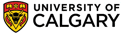Chapter 7 : StereochemistryFischer Projections

Fischer Projections are abbreviated structural forms that allow one to convey valuable stereochemical information to a chemist (or biochemist) without them having to draw a more detailed 3D structural representation of the molecule. These representations are only used for molecules that contain chirality centers, where the chirality centers are represented as simple crosses, e.g.The true 3D information present in a Fischer projection can be "extracted" by considering the complete 3D representation wedges and hashes which is built from the Fischer diagram knowing the convention that horizontal lines represent bonds coming out of the plane of the paper and vertical lines represent bonds going behind the plane of the paper. (This is the key to "decoding" Fischer diagrams).Memory Aid ? (this one works for me 100% of the time!) A student once told me that she remembered the relative arrangement of the bonds by the fact that the horizontal bonds were coming out to hug her ! (note: I was never a fan of Fischer diagrams because I had a hard time remembering this convention which had no logical basis, but with a hug to remember, it's not a problem).

When relating one Fischer projection to another, it's important to realise that it may only be manipulated within the 2D plane in which it is drawn (that is, it may not be arbitrarily rotated within 3D space), and even then, it can only rotated in the plane it is drawn (2D) by 180oA B
Why can't you rotate it 90o?  A 90o rotation is equivalent to breaking bonds and exchanging two groups, which would result in the formation of the other enantiomer.CAUTION Fischer projections are often confused with simpler Lewis diagrams.  Lewis diagrams, however, are not intended to give any stereochemical information!

Fischer projections a can also be used to represent molecules with more than one chirality center.If a Fischer projection of this type can be divided into two halves that are mirror images than the molecule may be identified as a meso isomer.Assignment of the configuration at a chirality center, in a Fischer projection, is based on the same Cahn-Ingold-Prelog rules.
The safest method for assigning the configuration in my opinion is probably to convert it to a wedge-hash diagram (as shown above)

Alternatively.... (and you need to know every step)....

• Identify the chirality centers (most commonly an sp3 C with 4 different groups attached).
• If the group of lowest priority is placed on a vertical line, this means the lowest priority group is already positioned away from you as if you were looking along the C-(4) s bond
• Now assess the direction of high to low priority (1 to 3)
• If this is clockwise, then the center is R (Latin: rectus = right)
• If this is counter clockwise, then it is S (Latin: sinister = left)
• If the group of lowest priority is placed on a horizontal line, this means the lowest priority group is actually positioned towards you (so we have to be very careful)
• Now assess the direction of high to low priority (1 to 3)
• If this is clockwise, then the center is R (Latin: rectus = right)
• If this is counter clockwise, then it is S (Latin: sinister = left)
• BUT NOW SWITCH THE ASSIGNMENT (it's like looking at a glass clock face from opposite sides)
Question : Consider the molecules A and B above. What are their configurations ? ANSWER?© Dr. Ian Hunt, Department of Chemistry